# 4.3 Graph with intercepts  (Page 2/5)

 Page 2 / 5

## Find the x - and y - intercepts from the equation of a line

Use the equation of the line. To find:

• the x - intercept of the line, let $y=0$ and solve for $x$ .
• the y - intercept of the line, let $x=0$ and solve for $y$ .

Find the intercepts of $2x+y=6$ .

## Solution

We will let $y=0$ to find the x - intercept, and let $x=0$ to find the y - intercept. We will fill in the table, which reminds us of what we need to find.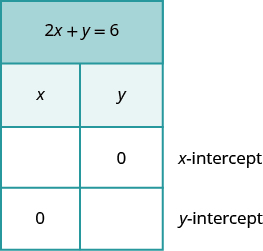To find the x - intercept, let $y=0$ .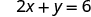Let y = 0.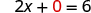Simplify.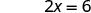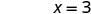The x -intercept is (3, 0) To find the y -intercept, let x = 0.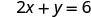Let x = 0.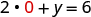Simplify.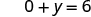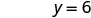The y -intercept is (0, 6)

The intercepts are the points $\left(3,0\right)$ and $\left(0,6\right)$ as shown in [link] .

 $2x+y=6$ $x$ $y$ 3 0 0 6

Find the intercepts of $3x+y=12.$

x - intercept: $\left(4,0\right)$ , y - intercept: $\left(0,12\right)$

Find the intercepts of $x+4y=8.$

x - intercept: $\left(8,0\right)$ , y - intercept: $\left(0,2\right)$

Find the intercepts of $4x–3y=12$ .

## Solution

 To find the x -intercept, let y = 0.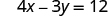Let y = 0.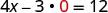Simplify.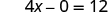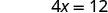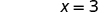The x -intercept is (3, 0) To find the y -intercept, let x = 0.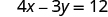Let x = 0.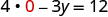Simplify.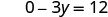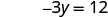The y -intercept is (0, −4)

The intercepts are the points (3, 0) and (0, −4) as shown in [link] .

 $4x-3y=12$ $x$ $y$ 3 0 0 $-4$

Find the intercepts of $3x–4y=12.$

x - intercept: $\left(4,0\right)$ , y - intercept: $\left(0,-3\right)$

Find the intercepts of $2x–4y=8.$

x - intercept: $\left(4,0\right)$ , y - intercept: $\left(0,-2\right)$

## Graph a line using the intercepts

To graph a linear equation by plotting points, you need to find three points whose coordinates are solutions to the equation. You can use the x - and y - intercepts as two of your three points. Find the intercepts, and then find a third point to ensure accuracy. Make sure the points line up—then draw the line. This method is often the quickest way to graph a line.

## How to graph a line using intercepts

Graph $–x+2y=6$ using the intercepts.

## Solution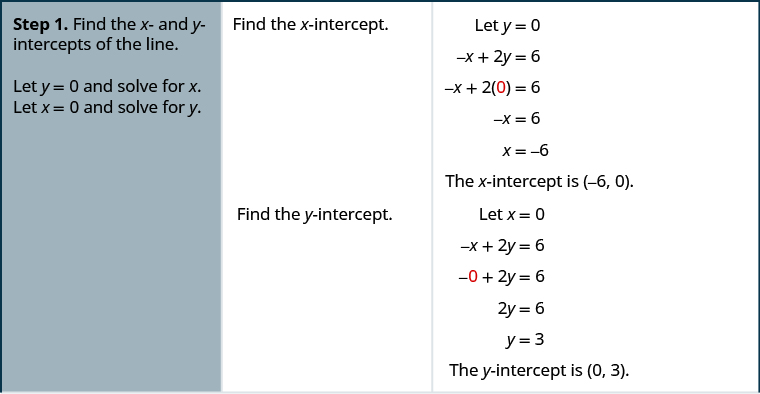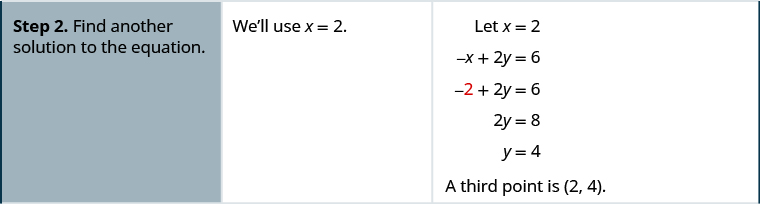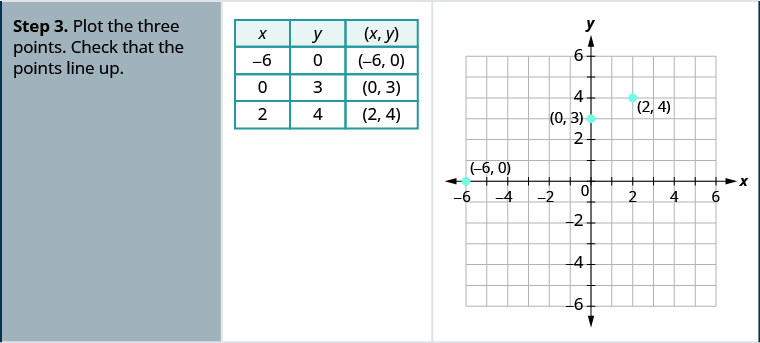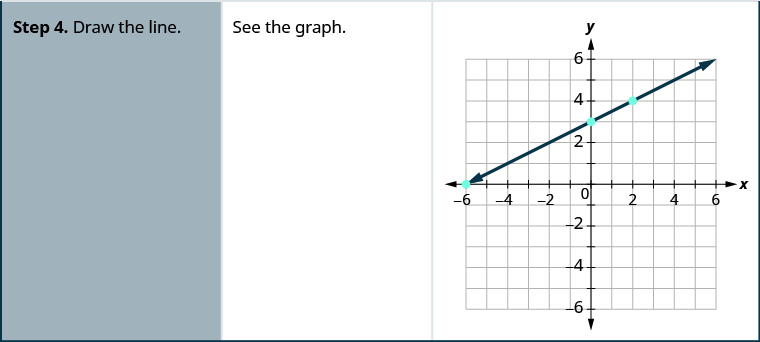Graph $x–2y=4$ using the intercepts.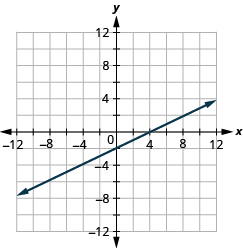Graph $–x+3y=6$ using the intercepts.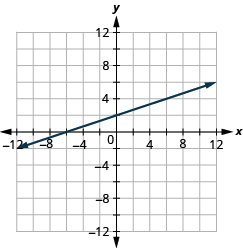The steps to graph a linear equation using the intercepts are summarized below.

## Graph a linear equation using the intercepts.

1. Find the x - and y - intercepts of the line.
• Let $y=0$ and solve for $x$
• Let $x=0$ and solve for $y$ .
2. Find a third solution to the equation.
3. Plot the three points and check that they line up.
4. Draw the line.

Graph $4x–3y=12$ using the intercepts.

## Solution

Find the intercepts and a third point.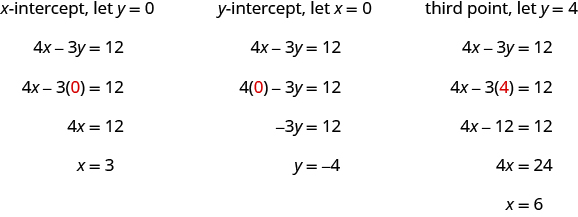We list the points in [link] and show the graph below.

 $4x-3y=12$ $x$ $y$ $\left(x,y\right)$ 3 0 $\left(3,0\right)$ 0 $-4$ $\left(0,-4\right)$ 6 4 $\left(6,4\right)$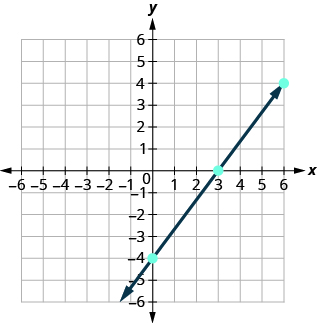Graph $5x–2y=10$ using the intercepts.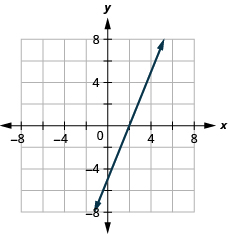Graph $3x–4y=12$ using the intercepts.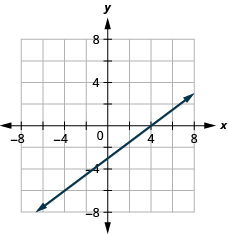Graph $y=5x$ using the intercepts.

## Solution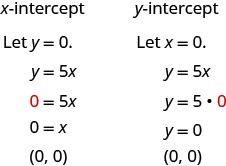This line has only one intercept. It is the point $\left(0,0\right)$ .

To ensure accuracy we need to plot three points. Since the x - and y - intercepts are the same point, we need two more points to graph the line.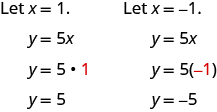See [link] .

 $y=5x$ $x$ $y$ $\left(x,y\right)$ 0 0 $\left(0,0\right)$ 1 5 $\left(1,5\right)$ $-1$ $-5$ $\left(-1,-5\right)$

Plot the three points, check that they line up, and draw the line.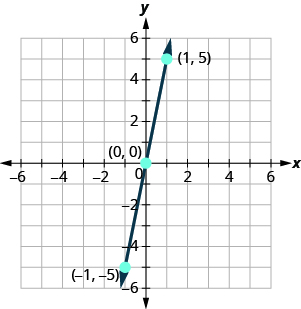Graph $y=4x$ using the intercepts.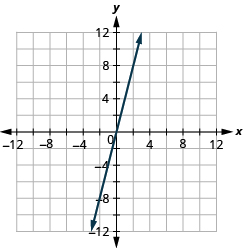Graph $y=\text{−}x$ the intercepts.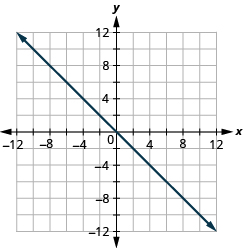## Key concepts

• Find the x - and y - Intercepts from the Equation of a Line
• Use the equation of the line to find the x - intercept of the line, let $y=0$ and solve for x .
• Use the equation of the line to find the y - intercept of the line, let $x=0$ and solve for y .
• Graph a Linear Equation using the Intercepts
1. Find the x - and y - intercepts of the line.
Let $y=0$ and solve for x .
Let $x=0$ and solve for y .
2. Find a third solution to the equation.
3. Plot the three points and then check that they line up.
4. Draw the line.

• Strategy for Choosing the Most Convenient Method to Graph a Line:
• Consider the form of the equation.
• If it only has one variable, it is a vertical or horizontal line.
$x=a$ is a vertical line passing through the x - axis at $a$
$y=b$ is a horizontal line passing through the y - axis at $b$ .
• If y is isolated on one side of the equation, graph by plotting points.
• Choose any three values for x and then solve for the corresponding y - values.
• If the equation is of the form $ax+by=c$ , find the intercepts. Find the x - and y - intercepts and then a third point.

## Practice makes perfect

Identify the x - and y - Intercepts on a Graph

In the following exercises, find the x - and y - intercepts on each graph.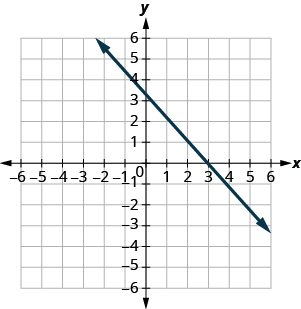$\left(3,0\right),\left(0,3\right)$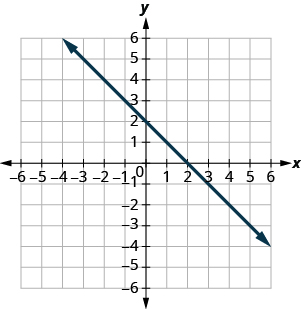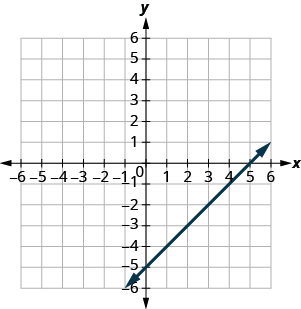$\left(5,0\right),\left(0,-5\right)$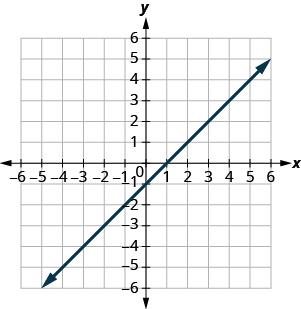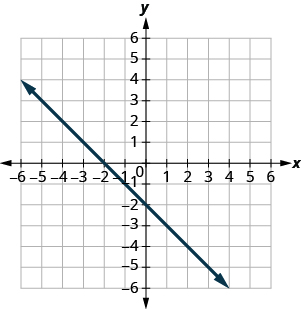$\left(-2,0\right),\left(0,-2\right)$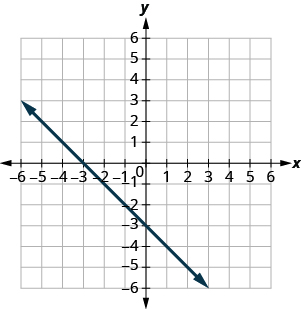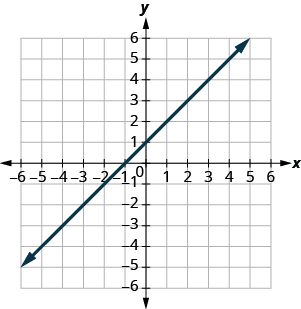$\left(-1,0\right),\left(0,1\right)$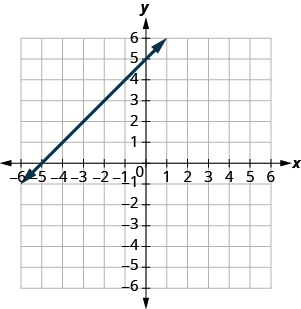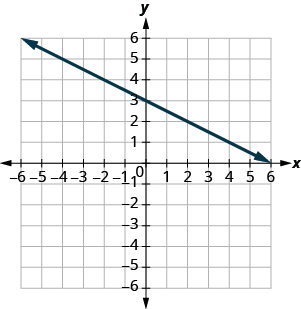$\left(6,0\right),\left(0,3\right)$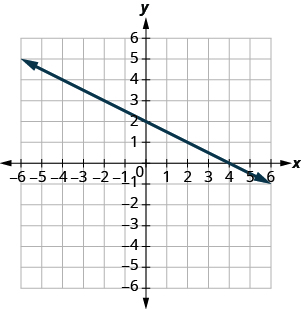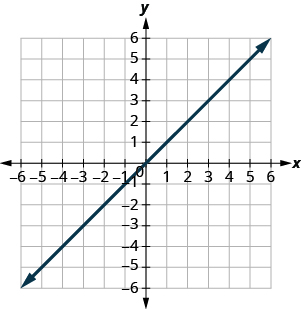$\left(0,0\right)$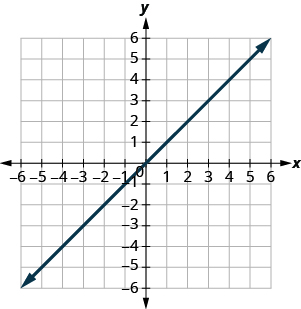Find the x - and y - Intercepts from an Equation of a Line

In the following exercises, find the intercepts for each equation.

$x+y=4$

$\left(4,0\right),\left(0,4\right)$

$x+y=3$

$x+y=-2$

$\left(-2,0\right),\left(0,-2\right)$

$x+y=-5$

$x–y=5$

$\left(5,0\right),\left(0,-5\right)$

$x–y=1$

$x–y=-3$

$\left(-3,0\right),\phantom{\rule{0.2em}{0ex}}\text{}\phantom{\rule{0.2em}{0ex}}\left(0,3\right)$

$x–y=-4$

$x+2y=8$

$\left(8,0\right),\left(0,4\right)$

$x+2y=10$

$3x+y=6$

$\left(2,0\right),\left(0,6\right)$

$3x+y=9$

$x–3y=12$

$\left(12,0\right),\left(0,-4\right)$

$x–2y=8$

$4x–y=8$

$\left(2,0\right),\left(0,-8\right)$

$5x–y=5$

$2x+5y=10$

$\left(5,0\right),\left(0,2\right)$

$2x+3y=6$

$3x–2y=12$

$\left(4,0\right),\left(0,-6\right)$

$3x–5y=30$

$y=\frac{1}{3}x+1$

$\left(3,0\right),\left(0,-1\right)$

$y=\frac{1}{4}x-1$

$y=\frac{1}{5}x+2$

$\left(-10,0\right),\left(0,2\right)$

$y=\frac{1}{3}x+4$

$y=3x$

$\left(0,0\right)$

$y=-2x$

$y=-4x$

$\left(0,0\right)$

$y=5x$

Graph a Line Using the Intercepts

In the following exercises, graph using the intercepts.

$–x+5y=10$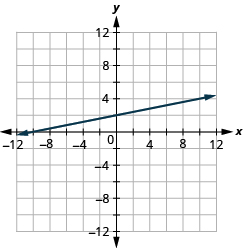$–x+4y=8$

$x+2y=4$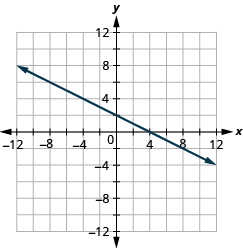$x+2y=6$

$x+y=2$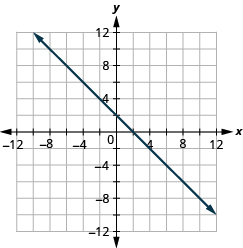$x+y=5$

$x+y=-3$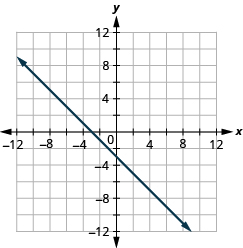$x+y=-1$

$x–y=1$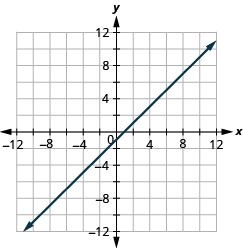$x–y=2$

$x–y=-4$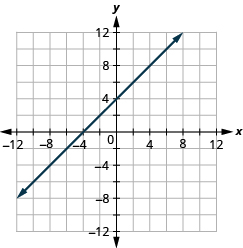$x–y=-3$

$4x+y=4$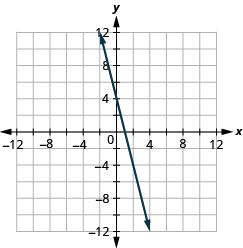$3x+y=3$

$2x+4y=12$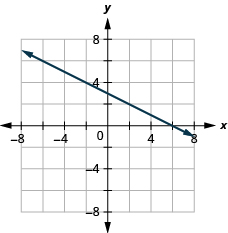$3x+2y=12$

$3x–2y=6$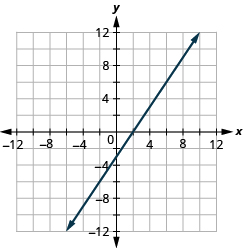$5x–2y=10$

$2x–5y=-20$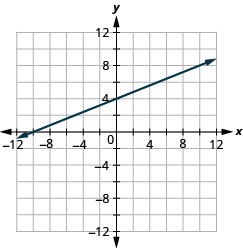$3x–4y=-12$

$3x–y=-6$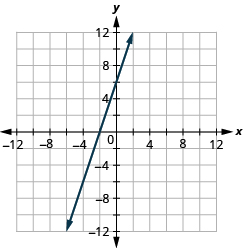$2x–y=-8$

$y=-2x$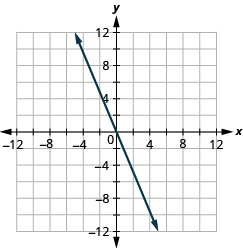$y=-4x$

$y=x$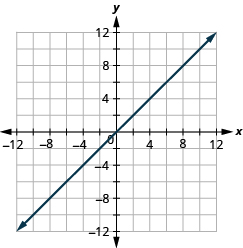$y=3x$

## Everyday math

Road trip. Damien is driving from Chicago to Denver, a distance of 1000 miles. The x - axis on the graph below shows the time in hours since Damien left Chicago. The y - axis represents the distance he has left to drive.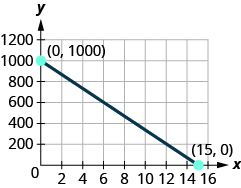1. Find the x - and y - intercepts.
2. Explain what the x - and y - intercepts mean for Damien.

$\left(0,1000\right),\left(15,0\right)$
At $\left(0,1000\right)$ , he has been gone 0 hours and has 1000 miles left. At $\left(15,0\right)$ , he has been gone 15 hours and has 0 miles left to go.

Road trip. Ozzie filled up the gas tank of his truck and headed out on a road trip. The x - axis on the graph below shows the number of miles Ozzie drove since filling up. The y - axis represents the number of gallons of gas in the truck’s gas tank.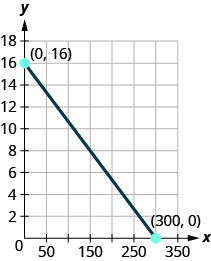1. Find the x - and y - intercepts.
2. Explain what the x - and y - intercepts mean for Ozzie.

## Writing exercises

How do you find the x - intercept of the graph of $3x–2y=6$ ?

Answers will vary.

Do you prefer to use the method of plotting points or the method using the intercepts to graph the equation $4x+y=-4$ ? Why?

Do you prefer to use the method of plotting points or the method using the intercepts to graph the equation $y=\frac{2}{3}x-2$ ? Why?

Answers will vary.

Do you prefer to use the method of plotting points or the method using the intercepts to graph the equation $y=6$ ? Why?

## Self check

After completing the exercises, use this checklist to evaluate your mastery of the objectives of this section.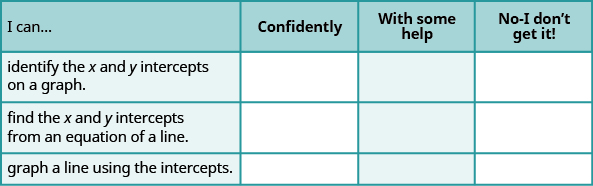What does this checklist tell you about your mastery of this section? What steps will you take to improve?

#### Questions & Answers

find the solution to the following functions, check your solutions by substitution. f(x)=x^2-17x+72
Carlos Reply
Aziza is solving this equation-2(1+x)=4x+10
Sechabe Reply
No. 3^32 -1 has exactly two divisors greater than 75 and less than 85 what is their product?
KAJAL Reply
x^2+7x-19=0 has Two solutions A and B give your answer to 3 decimal places
Adedamola Reply
please the answer to the example exercise
Patricia Reply
3. When Jenna spent 10 minutes on the elliptical trainer and then did circuit training for20 minutes, her fitness app says she burned 278 calories. When she spent 20 minutes onthe elliptical trainer and 30 minutes circuit training she burned 473 calories. How manycalories does she burn for each minute on the elliptical trainer? How many calories doesshe burn for each minute of circuit training?
Edwin Reply
.473
Angelita
?
Angelita
John left his house in Irvine at 8:35 am to drive to a meeting in Los Angeles, 45 miles away. He arrived at the meeting at 9:50. At 3:30 pm, he left the meeting and drove home. He arrived home at 5:18.
DaYoungan Reply
p-2/3=5/6 how do I solve it with explanation pls
Adedamola Reply
P=3/2
Vanarith
1/2p2-2/3p=5p/6
James
don't understand answer
Cindy
4.5
Ruth
is y=7/5 a solution of 5y+3=10y-4
Adedamola Reply
yes
James
don't understand answer
Cindy
Lucinda has a pocketful of dimes and quarters with a value of $6.20. The number of dimes is 18 more than 3 times the number of quarters. How many dimes and how many quarters does Lucinda have? Rhonda Reply Find an equation for the line that passes through the point P ( 0 , − 4 ) and has a slope 8/9 . Gabriel Reply is that a negative 4 or positive 4? Felix y = mx + b Felix if negative -4, then -4=8/9(0) + b Felix -4=b Felix if positive 4, then 4=b Felix then plug in y=8/9x - 4 or y=8/9x+4 Felix Macario is making 12 pounds of nut mixture with macadamia nuts and almonds. macadamia nuts cost$9 per pound and almonds cost $5.25 per pound. how many pounds of macadamia nuts and how many pounds of almonds should macario use for the mixture to cost$6.50 per pound to make?
Cherry Reply
Nga and Lauren bought a chest at a flea market for $50. They re-finished it and then added a 350 % mark - up Makaila Reply$1750
Cindy
the sum of two Numbers is 19 and their difference is 15
Abdulai Reply
2, 17
Jose
interesting
saw
4,2
Cindy
Felecia left her home to visit her daughter, driving 45mph. Her husband waited for the dog sitter to arrive and left home 20 minutes, or 13 hour later. He drove 55mph to catch up to Felecia. How long before he reaches her?
Rafi Reply
hola saben como aser un valor de la expresión
NAILEA

### Read also:

#### Get Jobilize Job Search Mobile App in your pocket Now!

Source:  OpenStax, Elementary algebra. OpenStax CNX. Jan 18, 2017 Download for free at http://cnx.org/content/col12116/1.2
Google Play and the Google Play logo are trademarks of Google Inc.

Notification Switch

Would you like to follow the 'Elementary algebra' conversation and receive update notifications?ByBy Richley CrapoBy Janet ForresterBy OpenStaxBy Edgar DelgadoBy Yasser IbrahimBy Anh DaoBy Maureen MillerByBy Richley Crapo